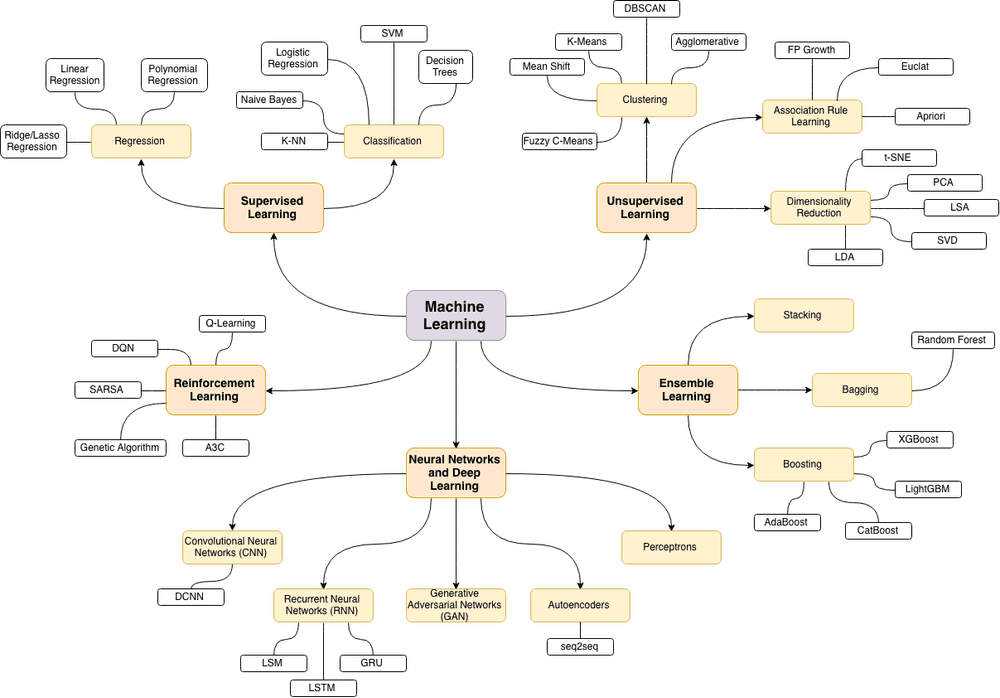# Homemade Machine Learning in Python

21 December, 2018The source of the following machine learning topics map is this wonderful blog post.

I’ve recently launched Homemade Machine Learning repository that contains examples of popular machine learning algorithms and approaches (like linear/logistic regressions, K-Means clustering, neural networks) implemented in Python with mathematics behind them being explained. Each algorithm has interactive Jupyter Notebook demo that allows you to play with training data, algorithms configurations and immediately see the results, charts and predictions right in your browser. In most cases the explanations are based on this great machine learning course by Andrew Ng.

The purpose of the repository was not to implement machine learning algorithms by using 3rd party library “one-liners” but rather to practice implementing these algorithms from scratch and get better understanding of the mathematics behind each algorithm. That’s why all algorithms implementations are called “homemade”.

The main Python libraries that are used there are NumPy and Pandas. These two are used for efficient matrix operations and for loading/parsing CSV datasets. When it comes to Jupyter Notebook demos then such libraries as Matplotlib and Plotly are being used for data visualizations.

Currently, the following topics have been covered:

## Regression: Linear Regression

In regression problems we do real value predictions. Basically we try to draw a line/plane/n-dimensional plane along the training examples.

Usage examples: stock price forecast, sales analysis, dependency of any number, etc.

## Classification: Logistic Regression

In classification problems we split input examples by certain characteristic.

Usage examples: spam-filters, language detection, finding similar documents, handwritten letters recognition, etc.

## Clustering: K-means Algorithm

In clustering problems we split the training examples by unknown characteristics. The algorithm itself decides what characteristic to use for splitting.

Usage examples: market segmentation, social networks analysis, organize computing clusters, astronomical data analysis, image compression, etc.

## Neural Networks: Multilayer Perceptron (MLP)

The neural network itself isn’t an algorithm, but rather a framework for many different machine learning algorithms to work together and process complex data inputs.

Usage examples: as a substitute of all other algorithms in general, image recognition, voice recognition, image processing (applying specific style), language translation, etc.

## Anomaly Detection: Gaussian Distribution

Anomaly detection (also outlier detection) is the identification of rare items, events or observations which raise suspicions by differing significantly from the majority of the data.

Usage examples: intrusion detection, fraud detection, system health monitoring, removing anomalous data from the dataset etc.

I hope you’ll find the repository useful. Either by playing with demos or by reading the math sections or by simply exploring the source code. Happy coding!